## Quantum Logic GatesDiving back into logic gates and quantum computing!

So last time, we talked about how ordinary, non-quantum, computers are built out of many, many logic gates. These logic gates defined operations on pairs of bits, like the and gate that follows this rule

1 and 1 = 1
0 and 1 = 0
1 and 0 = 0
0 and 0 = 0

They’re called logic gates because if you think of “1” as “true” and “0” as “false” then they look like logical ideas like “a and b are true if both a and b are true”. Combine enough of these logic gates together and you’ve got all the operations on numbers that a computer needs to function.

Now we get to the punchline of this three part story: how quantum logic gates, things that can be used to build up all the operations you need for a quantum computer, are different than ordinary logic gates.

So recalling a couple of issues ago, when we covered the basic idea of quantum computers, we said that a quantum bit—qbit—held data that could be used to compute the probability the qbit returns 1 or 0 when read. Now it’s finally time to talk about what that data even is. Surprise: it’s a point on a circle. The next part is going to involve a little bit of mathematics: namely the Pythagorean theorem and the square root operation. If you haven’t seen these things before, that’s okay, you can just follow along to get the idea!

Mathematical Aside:

To explain how “a wave that can be used to calculate probability”, which is what we’ve called qbits before, could be thought of as a point on a circle we need to use Pythagoras’s theorem!

So the Pythagorean theorem says that, for a circle of radius 1, if you take any point on the circle (x,y) then

x^2 + y^2 =1

Okay, well here’s the other fun little bit: when we talk about probability we generally deal with numbers that range from 0 to 1, where 1 means it’s 100% going to happen and 0 means 0% chance of it happening. If something is 50% likely, you’d write that as 0.5. This means that

(probability the qbit is 0) + (probability the qbit is 1) = 1

Okay, if we compare this to the above we get something kinda funny!

x^2 = probability the qbit is 0
y^2 = probability the qbit is 1

This means we can relate the points x and y to the probabilities. That means that every single point on the circle corresponds to some possible qbit state.

Mathematical aside over!

If the data in a qbit is a point on a circle, rather than a 1 or a 0, then what is a qgate? Well, a quantum gate is just a rotation of the circle. So to follow this, imagine drawing a point on the top of the circle. In our understanding of points as qbit data, then we know that this point means “the qbit is definitely 1”. If we rotate it 45 degrees clockwise, then we get the new state “the qbit is equally likely to be 1 or 0”. If we rotate it 45 degrees further then we get “the qbit is definitely zero”.

Now, what starts to get impressive is when we add multiple qbits. If you have two qbits, then qgates become rotating spheres in three-dimensions! If you have three qbits then qgates become rotating…uhh…well we don’t have a good word for dimensions higher than three but they’re like spheres but with more coordinate axes. So while a single ordinary logic gate can just operate on a few ones and zeros at a time quantum gates can do these big complicated things on higher-dimensional shapes you can’t even picture!

The other cool thing about quantum gates is that this means that you can always reverse the operation, the same way you’d reverse rotating a circle or a ball. You just go back the other way! You can’t do that with ordinary logic gates like and.

So all this being said, there’s one way you can start playing with these ideas and defining quantum gates and that’s with the Quantum JavaScript site.

Here you can build really simple gates out of pieces and then see how they change the probabilities. But, really, the main lesson from all this is that quantum gates are really fascinating!

#### Quantum logic gate

https://en.wikipedia.org/wiki/Quantum_logic_gate

#### demystifying quantum gates

https://towardsdatascience.com/demystifying-quantum-gates-one-qubit-at-a-time-54404ed80640

#### Pythagorean Theorem

https://en.wikipedia.org/wiki/Pythagorean_theorem

#### Pythagoras

https://www.mathsisfun.com/pythagoras.html

#### Introduction to logic gates

https://www.electronicshub.org/introduction-to-logic-gates/

#### Logic gates

https://mommycomputersciencecamp.com/logic-gates-b751f134053d

#### logic gate facts for kids

https://wiki.kidzsearch.com/wiki/Logic_gate

#### Probability

https://en.wikipedia.org/wiki/Probability

#### Probability line

https://www.mathsisfun.com/probability_line.html

#### Lessons plans – probability

https://www.mensaforkids.org/teach/lesson-plans/probably-probability/

#### Simple logic gates

https://www.instructables.com/Simple-Logic-Gates-and-Circuits/#:~:text=Simple%20Logic%20Gates%20and%20Circuits%201%20AND%20Gates.,many%20different%20ways.%20…%205%20Final%20Example.%20

#### Logic gates

https://www.javatpoint.com/logic-gates

## Author

•Clarissa has worked in mathematics, physics, and computer science research but spends much of her time now trying to make computer science education accessible to a broader audience.

### Also In The August 2021 IssueWhenever you're online there are always some basic rules you should be aware.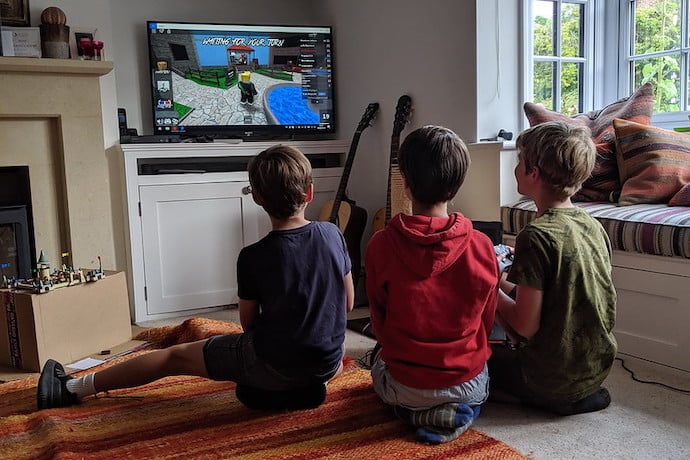Read our guide on how to set up a Roblox Account.English is a difficult quirky language to learn. Noah Text patterns help people learn English more easily.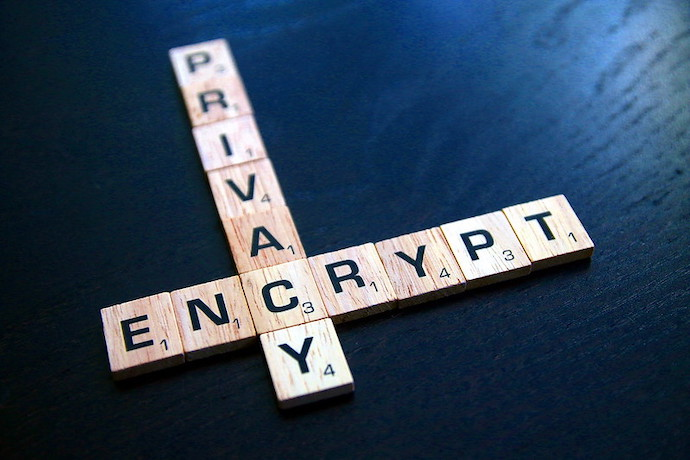Do you have a secret you want to pass to your friend? Try using a 'one time pad' code!Sadly your computer doesn't have a tiny sheep in it, but what it does have is pretty neat!Learn how the IoT is helping with COVID-19 vaccine!Make your own lava block with this fun project!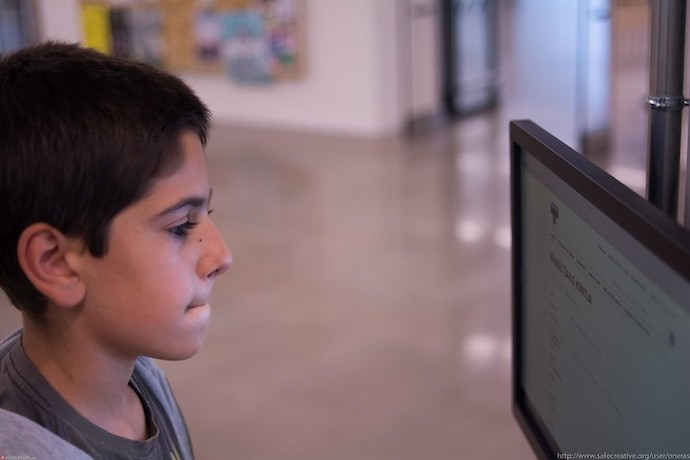Learn how to make music and videos with your friends using Estuary!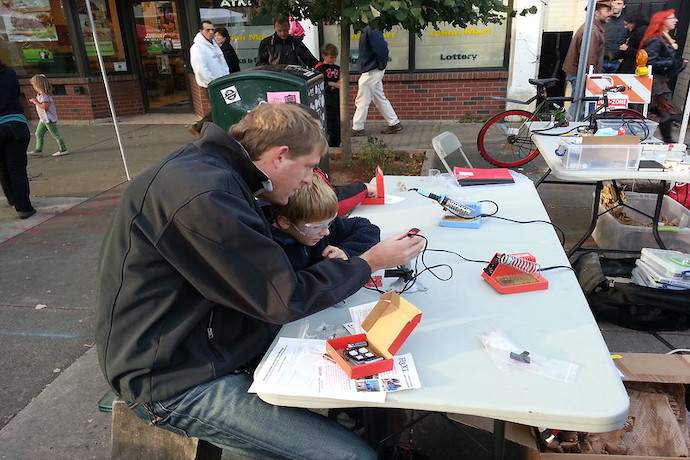Keep developing your soldering skills with this fun activity!Learn about the history of the world wide web and how browsers have evolved over time.Problems software programmers can do to practice their skills, from beginner to advanced.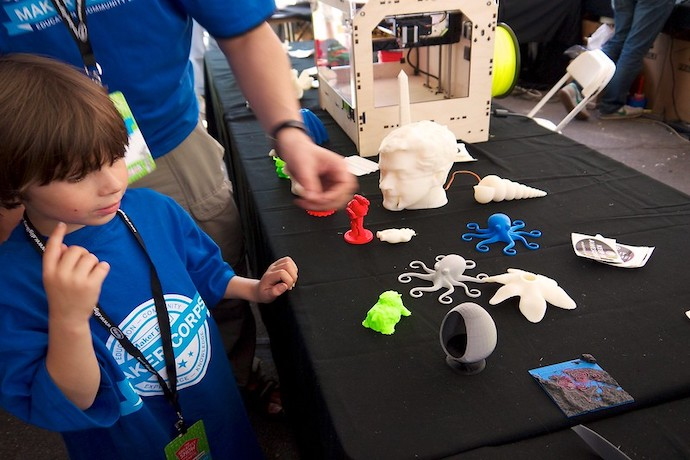Discussing how framing failure as part of a greater game can help young designers.Learn about the first time CGI was ever used for animation!Diving back into logic gates and quantum computing!The Learnmore links from the August 2021 stories, all in one place.Interesting stories about computer science, software programming, and technology.Interested but not ready to subscribe? Sign-up for our free monthly email newsletter with curated site content and a new issue email announcement that we send every two months.

No, thanks!